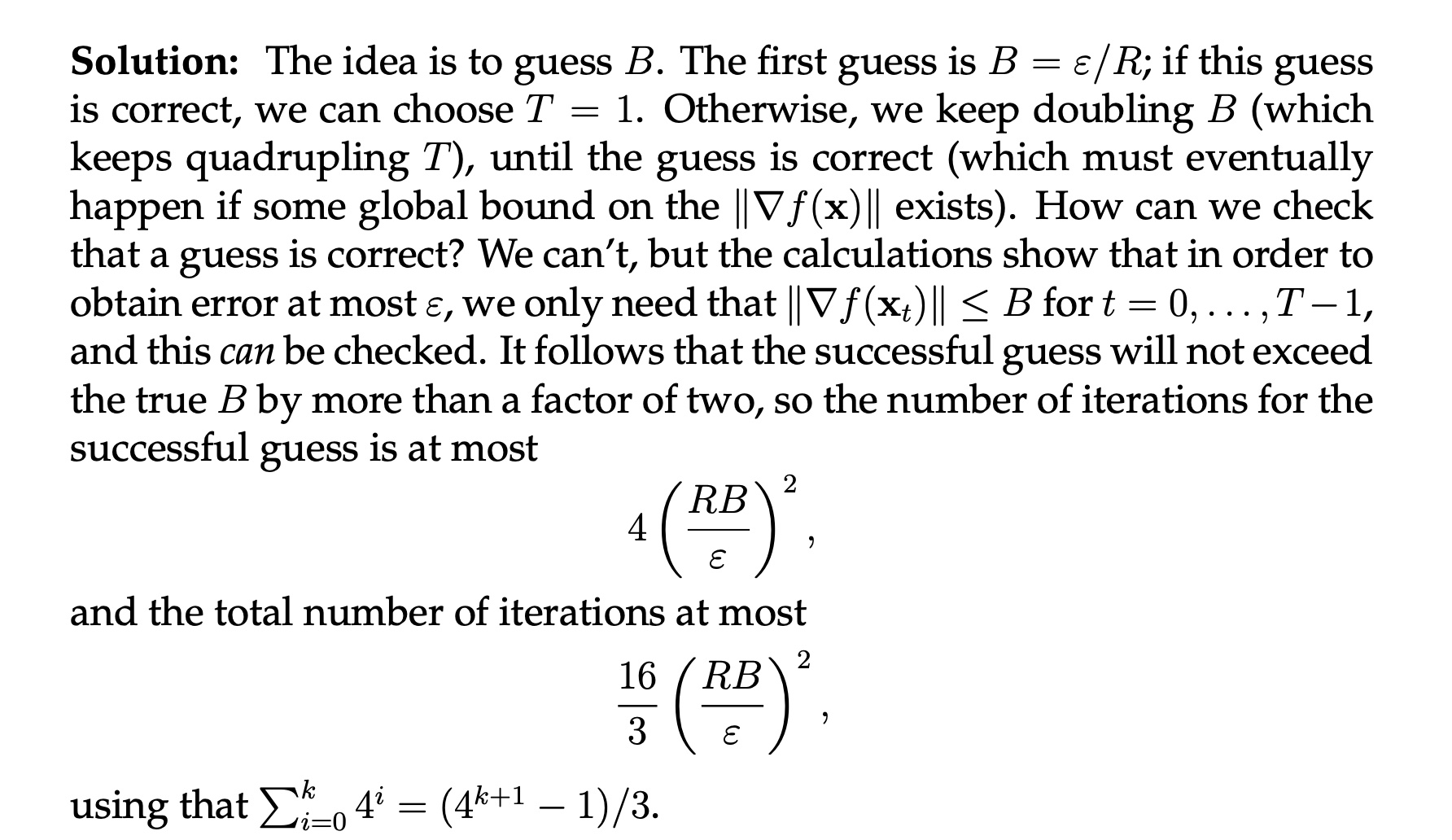### Exercise 15 - Question on the total number of iterationsI don't understand the last step in this calculation. How do we apply the sum of 4^i formula to get a constant 16/3 factor?

Thanks!

Top comment

for every guess of B until (and including) the successful one, you still have to run the optimization algorithm. each run takes (B'R/eps)^2 steps, where B' is your guess. the first guess is B'=eps/R, so one step. the second guess is twice that (doubles each time), so 4 steps (quadruples each time). that's how the sum 4^i series comes in. for the last 4^i term we just plug in 4 (BR/eps)^2, which comes from 2 * true B

Page 1 of 1